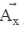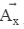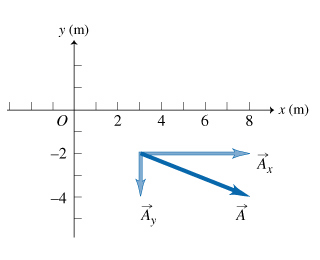# Problem: Tactics Box: Determining the components of a vector1. The absolute value |Ax| of the x component Ax is the magnitude of the component vector .2. The sign of Ax is positive if points in the positive x direction; it is negative if points in the negative x direction.3. The y component is determined similarly.A. What is the magnitude of the component vector Ax shown in the figure?Express your answer in meters to one significant figure.B. What is the sign of the y-component Ay of vector A shown in the figure?

###### FREE Expert Solution

A.

Ax = xhead - xtail = 8 - 3 = 5 m

100% (418 ratings)###### Problem Details

Tactics Box: Determining the components of a vector

1. The absolute value |Ax| of the x component Ax is the magnitude of the component vector.

2. The sign of Ax is positive ifpoints in the positive x direction; it is negative ifpoints in the negative x direction.

3. The y component is determined similarly.

A. What is the magnitude of the component vector Ax shown in the figure?